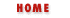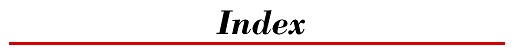Symbols | A | B | C | D | E | F | G | H | I | J | K | L | M | N | O | P | Q | R | S | T | U | V | W | X | Y

# Symbols and Numbers

/2 constant : Math.SQRT1_2
constant : Math.SQRT2
& (bitwise and) operator : Bitwise And...
&& (logical and) operator
Miscellaneous Differences
Logical And...
&{} for entities
Core Language Changes
JavaScript Entities
' (apostrophe)
String Literals
Escape Sequences in String Literals
* (multiplication) operator : Multiplication (*)
\ (backslash) : Escape Sequences in String Literals
!= (inequality) operator : Inequality (!=)
! (logical not) operator : Logical Not (!)
[] (brackets)
Arrays
Array and Object Access Operators
for...in
Objects as Associative Arrays
Conversions to Objects
^ (bitwise exclusive or) operator : Bitwise Xor (^)
, (comma) operator
The Comma Operator (,)
for
{} (curly braces)
Compound Statements
function
\$ (dollar sign) : Identifiers
. (dot) operator
Array and Object Access Operators
Arrays in Navigator 2.0
Conversions to Objects
XX_DQUOTE_XX (double quote) : String Literals
= (assignment) operator
Equality (==)
Assignment Operators
The assign() Method
combined with operations : Assignment with Operation
== (equality) operator : Equality (==)
> (greater than) operator
Greater Than (>)
String Operators
>= (greater than or equal) operator
Greater Than or Equal (>=)
String Operators
>> (shift right with sign) operator : Shift Right with Sign (>>)
>>> (shift right zero fill) operator : Shift Right Zero Fill (>>>)
- (minus) operator : Subtraction (-)
- (negation) operator : Unary Negation (-)
-- (decrement) operator : Decrement (--)
< (less than) operator
Less Than (<)
String Operators
<!-- --> (comment tags)
Hiding Scripts from Old Browsers
<= (less than or equal) operator
Less Than or Equal (<=)
String Operators
<< (shift left) operator : Shift Left (<<)
() (parentheses)
Functions
Objects
Operator Precedence
Function Call Operator
Defining and Invoking Functions
% (modulo) operator : Modulo (%)
| (bitwise or) operator : Bitwise Or (|)
|| (logical or) operator
Miscellaneous Differences
Logical Or (||)
+ (plus/concatenate) operator
Strings
String Operators
Conversions to Strings
Workarounds for Navigator 2.0
++ (increment) operator : Increment (++)
?: (conditional) operator : The Conditional Operator (?:)
; (semicolon)
Optional Semicolons
Statements
XX_SQUOTE_XX (single quote) : String Literals
/ (division) operator : Division (/)